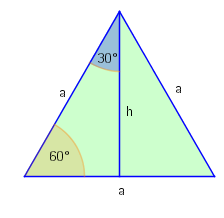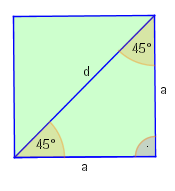# What is the value of cos 1

## Special values ​​of the trigonometric functions

For selected angles, the values ​​of the trigonometric functions can also be represented as root expressions.

### Table 7CGF

Degree30°45°60°90°
wheel 0
sinx021​21​2​21​3​1
cosx121​3​21​2​21​0
tanx031​3​13​ n.def.
cotx n.def. 3​131​3​0

### DerivationIn an equilateral triangle we find the angles 30 ° and 60 °. According to the Pythagorasa theorem, it applies2 = h2 + (2a) 2, from which h = 23 a follows. With the definition of sine and cosine we get:

sin30 ° = cos60 ° = a2a = 21

and

cos30 ° = sin60 ° = ah = a23 a = 21 3In a square with side length a, according to the Pythagorean theorem, the length of the diagonal end = 2 a. With this we get:

sin45 ° = cos45 ° = 2 aa = 21 2.

Every science needs mathematics, mathematics does not need any.

Jacob I. Bernoulli